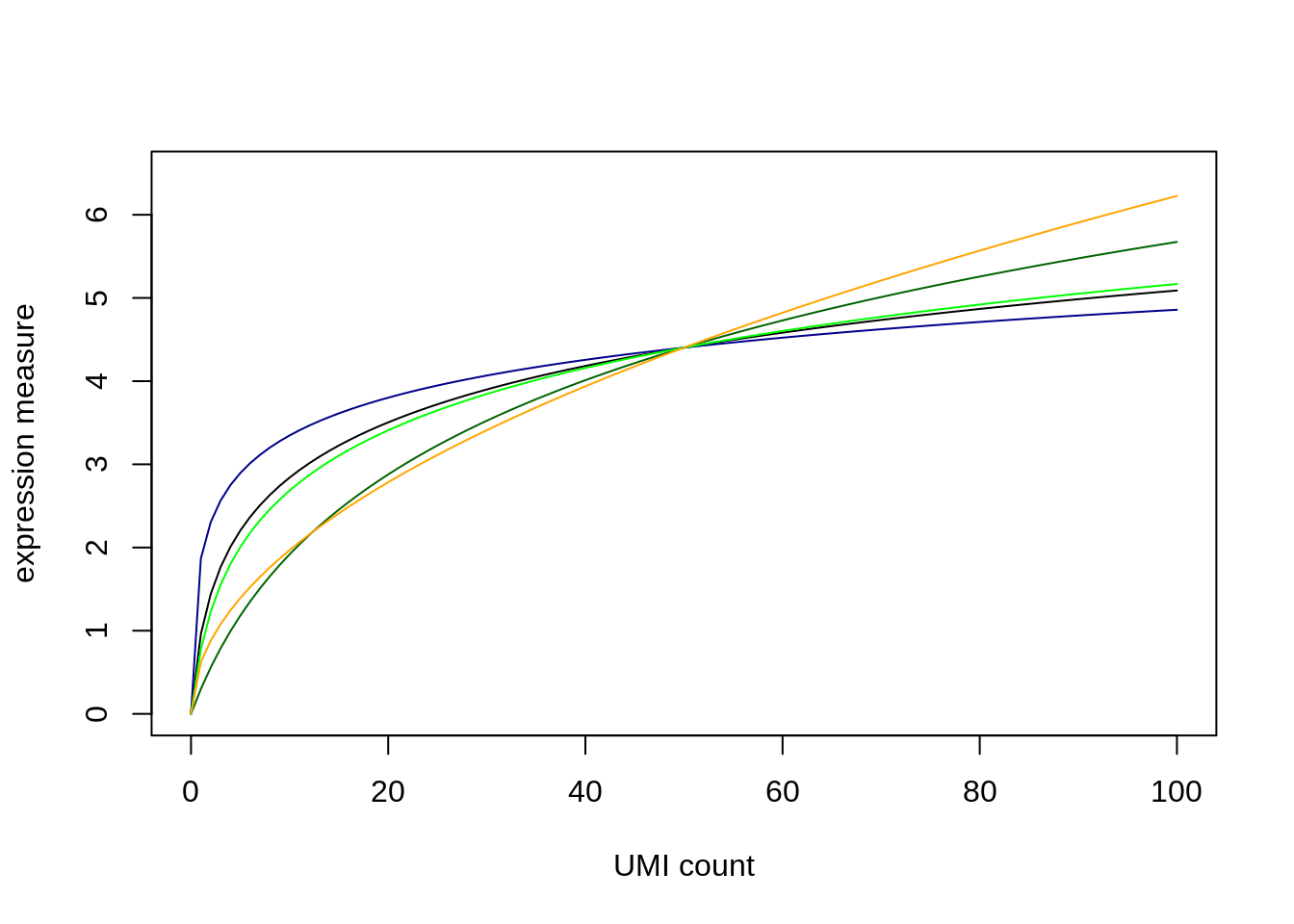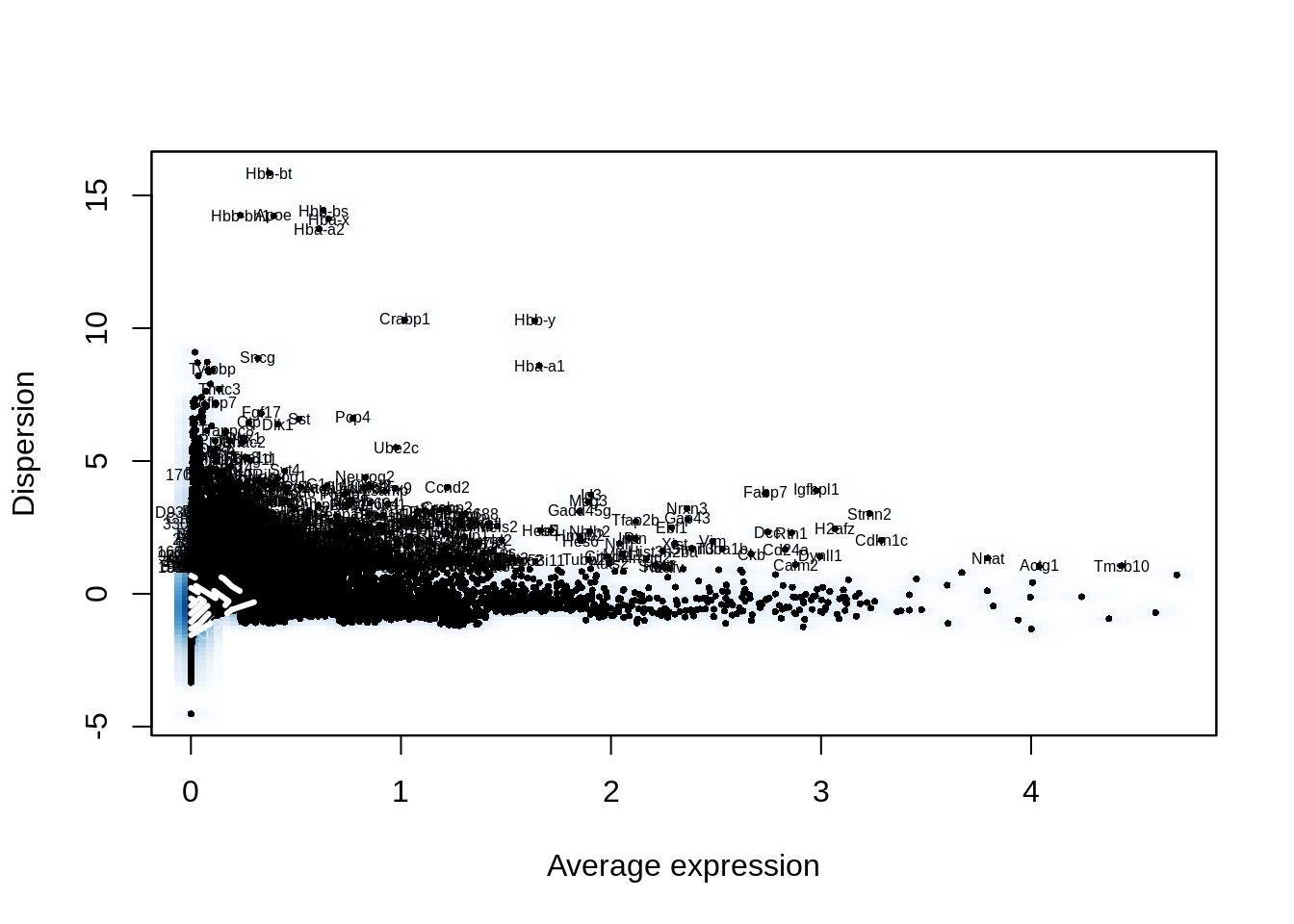This document compares two approaches to analyse single-cell data: First, we use Seurat, and then we perform all steps using only basic R, in order to be able to study and modify each step. I have presented it at the Single Cell Center Heidelberg meeting in Mar 2019.

## Example data

As example data, I use the study on the development of the mouse brain by Carter et al.: “A single-cell transcriptional atlas of the developing murine cerebellum”, Current Biology 28:2910 (2018).

Specifically, I will use their sample E13a, i.e., which comprises cells from the cerebellum of a mouse embryo at day 13.5. The “a” means that it is the first of their two replicates.

## Seurat analysis

I first perform a standard analysis with Seurat.

library( Seurat )

Kevin has downloaded the bam files from the European Nucleotide Archive (accession PRJEB23051) and run them again through CellRanger. In the next line, I read in the output from CellRanger. If you want to rerun this script yourself, you can find the three CellRanger output files for sample E13a here.

seu <- Read10X("E13_A/")

Now I create a Seurat object, keeping only the genes that are expressed in at least 3 cells, and only those cells expressing at least 1000 genes.

seu <- CreateSeuratObject( seu, min.cells = 3, min.genes=1000 )

I normalize the data. This performs, by default, the transformation $$y_{ij} = \log\left( 1 + 10^4 \frac{k_{ij}}{\sum_{i'}k_{i'k}}\right)$$ (where $$k_{ij}$$ is the UMI count for gene $$i$$ in cell $$j$$).

seu <- NormalizeData( seu )

A short detour here on the topic of normalization:

The median UMI count in our cells is

median_umi_count_per_cell <- median( colSums(seu@raw.data) )
median_umi_count_per_cell
##  6199

Here is a plot showing how the transformation changes UMI counts for such a “median” cell

plot( 0:100, log( 1 + 1e4 * (0:100) / median_umi_count_per_cell ),
type = "l", xlab="UMI count", ylab="expression measure", ylim = c(0, 6.5) )

meet_at_50 <- function(y)
y / y * log( 1 + 1e4 * 50 / median_umi_count_per_cell )

lines( 0:100, meet_at_50( log( 1 + 1e3 * (0:100) / median_umi_count_per_cell ) ), type = "l", col="darkgreen" )
lines( 0:100, meet_at_50( log( 1 + 1e5 * (0:100) / median_umi_count_per_cell ) ), type = "l", col="darkblue" )
lines( 0:100, meet_at_50( log10( 1 + (0:100) ) ), type = "l", col="green" )
lines( 0:100, meet_at_50( sqrt( (0:100) / median_umi_count_per_cell ) ), type = "l", col="orange" )The black line above is Seurat’s default normalization. For future reference, I have also included the transformation if one changes Seurat’s scaling factor from the default $$10^4$$ (black) to $$10^3$$ (dark green) or $$10^5$$ (dark blue). Light green is the often used simple $$y_{ij} = \log_{10}(1+k_{ij})$$ and orange is the square-root transform $$y_{ij} = \sqrt{ {k_{ij}}/{\sum_{i'}k_{i'k}}}$$. I have rescaled these extra lines so that they all meet at 50.

Continuing with the standard Seuirat work-flow: Find the variable genes

seu <- FindVariableGenes( seu )Scale and center the data and regress out UMI totals

seu <- ScaleData( seu, vars.to.regress = "nUMI" )
## Regressing out: nUMI
##
## Time Elapsed:  29.526376247406 secs
## Scaling data matrix

Run a PCA to reduce the data

seu <- RunPCA( seu, do.print = FALSE )

Perform Louvain clustering

seu <- FindClusters( seu, print.output = FALSE, save.SNN = TRUE )

Get an embedding. I use UMAP. By default, RunUMAP only uses the first 5 PCS, but this gives quite unsatisfactory results. Hence, I use a few more, otherwise it get’s too blurry.

seu <- RunUMAP( seu, dims.use=1:20 )

Plot the embedding, and highlight the Louvain clusters:

DimPlot( seu, "umap" )